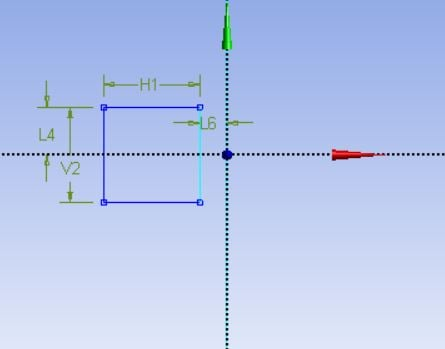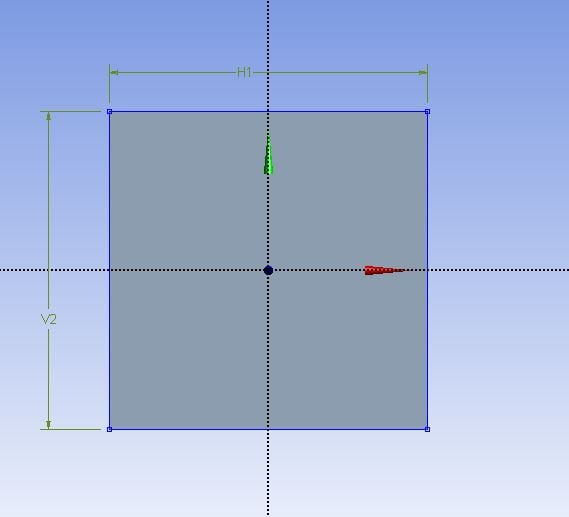## Fluids

•vwiegmann
Subscriber

Hi everyone,

I intend to rotate my model around the origin with a radius of 25 mm, while rotating the model around its center so that it always faces the same way. The UDF that I have (see below) works well for round models, but I have trouble generating the same motion with my square model (model rotates around its center, but only travels along the x-axis).
Do any of you know why this could be the case?
I use ANSYS Fluent; after compiling the UDF, I select it under mesh motion in the Cell Zone Boundaries tab.

Any help is greatly appreciated!!

•Rob
Ansys Employee

Can you check the round model was working correctly? The shape shouldn't have an effect so I wonder if you're just not seeing the issue in the earlier test.

As staff we can't get too involved, so we're reliant on the community to debug cases and code.

•vwiegmann
Subscriber

Thank you very much for your help and time. I have tried it with the round version, which worked well (cylinder rotates around the origin). The same code with a square well results in above motion. This would suggest a problem with the model. I have since tried two things:

1) The distance between the center of the model and the origin is equal to the orbital diameter of the rotation: model rotates around its center, but only travels along the x-axis.

2) Center of the model lies on the origin: model rotates around its center, but only travels along the y-axis.

Not sure what to make of this at the moment. Any input is greatly appreciated!

•Keyur Kanade
Ansys Employee

Hello,

As rwoolhou, as ANSYS employee we can not download attachments and can not debug cases and code.

Can you please insert some images with more description.

Thanks!

Regards,

Keyur

•vwiegmann
Subscriber

Hey Keyur,

Here are some configurations that I have tested with below UDF:This is the footprint of the model. It is supposed to rotate around the origin but only rotates around itself while moving along the x-axis. When changing the 'rdar' to '0' in the UDF, the rotation around the origin will be accurate, but the rotation around the centre of the model is missing.Only rotates around itself while moving along the y-axis.

UDF:

#include "udf.h"
#define PI 3.14592654
DEFINE_ZONE_MOTION(rotation, omega, axis, origin, velocity, time, dtime)
{
real theta0, theta1, omegac, omegar, rdar, ox, oy;
omegar = -20.94;
omegac = -omegar;
theta0 = PI;
theta1 = omegac*(time+dtime);
*omega = omegar;
rdar = 0.0125;
ox = rdar*cos(theta0+theta1);
oy = rdar*sin(theta0+theta1);
origin  = ox;
origin  = oy;
origin = (0,0);
}

•Keyur Kanade
Ansys Employee

You can find its example in help.

Regards,

Keyur

•vwiegmann
Subscriber

Thank you very much Keyur. If I understand correctly, though, DEFINE_CG_MOTION would only let me rotate the model around the origin. What I intend to do is rotate the model around the origin while at the same time rotating it around its center, so that the square always faces the same direction during the rotation. Is that possible with DEFINE_CG_MOTION?

•Antoni
Subscriber

I have the same problem here, have you found the answer?

•Rob